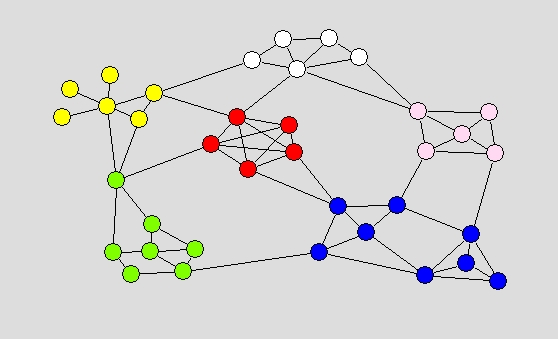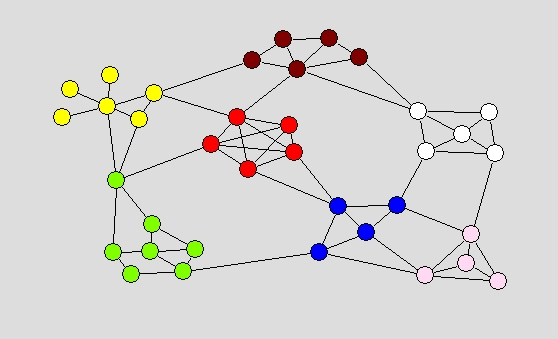### Simple small example

Lets first take a simple constructed small network shr1.net and compare results obtained using Louvain method and VOS Clustering with different resolution parameters (r).

#### Resolution parameter r=1

For resolution parameter r=1 Louvain method and VOS Clustering find almost the same partitions into 6 clusters. The difference is in position of a single vertex (belonging to 'yellow' cluster in one and 'green' cluster in other partition). Visually both partitions seems equally good, but looking at Q and VOSQ values there are small differences that make one partition a bit better than the other.

 Best partition according to Louvain method Best partition according to VOS Clustering Q(r=1)=0.633651, VOSQ(r=1)=0.701099 VOSQ(r=1)=0.708359, Q(r=1)=0.632029#### Resolution parameter r=1.5

By setting resolution parameter to 1.5 both methods splits the 'blue' cluster into two smaller clusters.

 Best partition according to Louvain method Best partition according to VOS Clustering Q(r=1.5)=0.546064, VOSQ(r=1.5)=0.631591 VOSQ(r=1.5)=0.638852, Q(r=1.5)=0.543631#### Resolution parameter r=0.2

By setting resolution parameter to 0.2 both methods find the same partition into 3 clusters.

 Best partition according to Louvain method and VOS Clustering Q(r=0.2)=0.827768, VOSQ(r=0.2)=0.855510